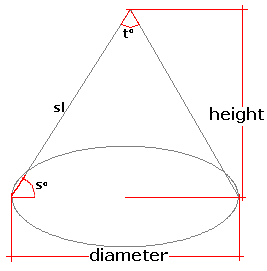Spike's Calculators

# Cone Volume and Area

Calculate the volume, surface area, slant height, and the slant angle of a right circular cone.This calculator works for any measurement unit. For example, if you calculate inches, inches will have to be used in all the length fields, and the result of the areas will be square inches; the volume results in cubic inches and length measurements in the starting unit, inches.

### Cone

Height of Cone #
Base Diameter #
Decimal Precision #

#### Results:

 Base Radius # Volume #³ Lateral Surface #² Base Area #² Surface Area #² Slant Length # Slant Angle ° Top Angle ° Surface to Volume Ratio sa:vol

#### Calculation

• the height of the cone
• the diameter @ the base of the cone
• decimal precision, number of digits after the decimal point

#### Results

• the radius of the base (starting unit)
• the volume of the cone volume (unit cubed)
• the lateral area of the cone (unit squared)
• the base (bottom) area of the cone (unit squared)
• the surface area of the cone (unit squared)
• slant length (starting unit)
• slant angle (degrees)
• top angle (degrees)
• surface area to volume ratio (decimal)
##### Formula
```r = B/2
V = 1/3Π(r)²H
LA = Π(r)√(r)²+H²)
BA = Π(r)²
SL = √(r)²+H²
SA = Πr(r+√(r)²+H²)
s = sin-1(H/SL)
t = sin-1(r/SL)
s/v = SA/V
V  the volume of the cone
Π = 3.14159265
B the base length
H the height of the cone
LA  the lateral area of the cone
BA  the base area of the cone
SA the surface  area of the cone
SL the length of the slant
s the angle of the slant
t the top angle
s/v is the surface area to volume ratio
```